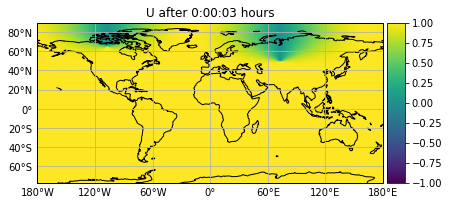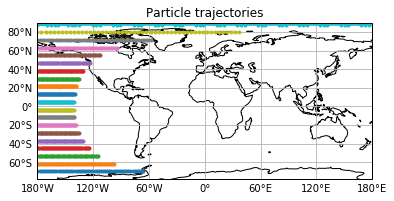## Tutorial on how to use Parcels on NEMO curvilinear grids¶

Parcels also supports curvilinear grids such as those used in the NEMO models.

We will be using the example data in the NemoCurvilinear_data/ directory. These fiels are a purely zonal flow on an aqua-planet (so zonal-velocity is 1 m/s and meridional-velocity is 0 m/s everywhere, and no land). However, because of the curvilinear grid, the U and V fields vary north of 20N.

In :
from parcels import FieldSet, ParticleSet, JITParticle, ParticleFile, plotTrajectoriesFile
import numpy as np
from datetime import timedelta as delta
%matplotlib inline


We can create a FieldSet just like we do for normal grids. Note that NEMO is discretised on a C-grid. U and V velocities are not located on the same nodes (see https://www.nemo-ocean.eu/doc/node19.html ).

 __V1__
|      |
U0     U1
|__V0__|

To interpolate U, V velocities on the C-grid, Parcels needs to read the f-nodes, which are located on the corners of the cells (for indexing details: https://www.nemo-ocean.eu/doc/img360.png ).

In :
data_path = 'NemoCurvilinear_data/'
filenames = {'U': {'lon': data_path + 'mesh_mask.nc4',
'data': data_path + 'U_purely_zonal-ORCA025_grid_U.nc4'},
'data': data_path + 'V_purely_zonal-ORCA025_grid_V.nc4'}}
variables = {'U': 'U',
'V': 'V'}
dimensions = {'lon': 'glamf', 'lat': 'gphif', 'time': 'time_counter'}
field_set = FieldSet.from_nemo(filenames, variables, dimensions, allow_time_extrapolation=True)

WARNING: Casting lon data to np.float32
WARNING: Casting lat data to np.float32
WARNING: Casting depth data to np.float32


And we can plot the U field.

In :
field_set.U.show()As you see above, the U field indeed is 1 m/s south of 20N, but varies with longitude and latitude north of that

Now we can run particles as normal. Parcels will take care to rotate the U and V fields

In :
# Start 20 particles on a meridional line at 180W
npart = 20
lonp = -180 * np.ones(npart)
latp = [i for i in np.linspace(-70, 88, npart)]

# Create a periodic boundary condition kernel
def periodicBC(particle, fieldset, time):
if particle.lon > 180:
particle.lon -= 360

pset = ParticleSet.from_list(field_set, JITParticle, lon=lonp, lat=latp)
pfile = ParticleFile("nemo_particles", pset, outputdt=delta(days=1))
pset.execute(kernels, runtime=delta(days=50), dt=delta(hours=6),
output_file=pfile)

INFO: Compiled JITParticleAdvectionRK4periodicBC ==> /var/folders/r2/8593q8z93kd7t4j9kbb_f7p00000gr/T/parcels-504/31a86092b3849f0d767018c4324d26a8.so
100% (4320000.0 of 4320000.0) |##########| Elapsed Time: 0:00:01 Time:  0:00:01


And then we can plot these trajectories. As expected, all trajectories go exactly zonal and due to the curvature of the earth, ones at higher latitude move more degrees eastward (even though the distance in km is equal for all particles)

In :
pfile.export()  # export the trajectory data to a netcdf file
plotTrajectoriesFile("nemo_particles.nc");